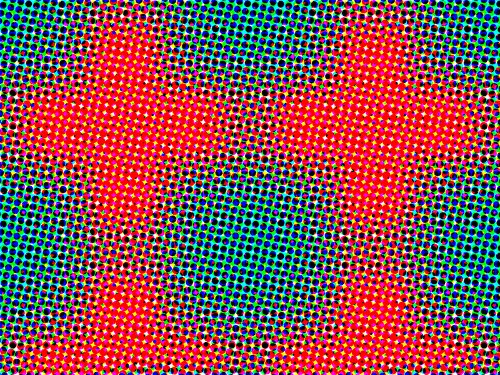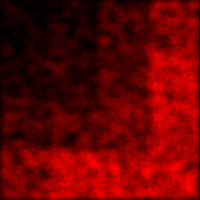# Generating Random Points from Arbitrary Distributions for 2D and UpI have already covered how to generate random numbers from arbitrary distributions in the one-dimensional case. Here we look at a generalisation of that method that works for higher dimensions.

The basic trick, while easy to understand, is hard to put in words (without reverting to mathematical equations). For two dimensions, we divide the plane into slices. Each slice is a 1D distribution. We also calculate a distribution from summing the frequencies in each slice. The latter distribution gives us one coordinate, and the appropriate slice to use. The distribution of that slice then gives the second coordinate. All distributions are put into inverse accumulative response curves as was done to generate one-dimensional random numbers. (You should review that before implementing the 2D case).

In more dimensions, we also slice the space up into 1D distributions. Sums of these give us more distributions, which we can sum again, and again, until we reach a single distribution. This is used for the first coordinate, and to determine which distribution to use for the next coordinate. This goes on, until a 1D slice gives us the final coordinate. Again, all distributions are converted to inverse accumulative response curves.

If the above is unclear, I hope the detailed description below clears things up.

## Two Dimensions

### Describe the Distribution

Divide the domain into a regular grid. Let us use the domain 10..40 x 10..40. This means, all the points we will generate will fall in the square between the lines x = 10, x = 40, y = 10 and y = 40.

For later use, we will need the minimum x-coordinate of the domain (x0 = 10), the width of the domain (w = x1 – x0 = 40 – 10 = 30).

Assign to each cell the number of random numbers that should be generated for that cell for any suitable total of points. You need not normalise these values.

For our example we will use the 4×4 grid shown below:

 1 2 4 8 2 3 5 11 4 5 7 11 8 11 11 11

For later use, we need the width of the grid (gw = 4).

The method described here won’t work if any of these values are 0.

### Calculate Cumulative Grids

Calculate the cumulative sums for the columns of the array.

 1 2 4 8 3 5 9 19 7 10 16 30 15 21 27 41

Calculate a cumulative sum for the last row

 15 36 63 104

### Construct Inverse Response Curves

For each column of cumulative sums, append a zero at the beginning, and create an inverse response curve as described for the one dimensional case. Thus the first columns inverse response curve will be created from 0, 1, 3, 7, 15. Call these curves cy … cy.

For the row of cumulative sums, append a zero at the beginning, and create an inverse response curve. Call this curve cx.

### Determine Random Point

You are now ready to generate random points from the specified distribution.

First, generate two uniform random numbers, urx and ury.

Use urx to do a lookup into curve c[x]. The result rx gives the x-coordinate of your non-uniform random number. Use this number to decide which column curve to use: subtract the minimum domain x-coordinate, divide it by the domains width, multiply it by the number of columns, and floor it to an integer.

ix = (rx – x0)/w * gw

Now use ury to do a lookup in cy[ix]. The result ry is the y-coordinate of the number.This image shows a plot of samples generated from the distribution specified above (normalised to fit in the image boundaries). Brighter areas indicate that more points occur in that region.

Visually, it looks like the sample mimics the original distribution well.

## N Dimensions

### Describe the Distribution

Divide the domain in a regular N-dimensional grid, and assign frequencies to cells in the grid. For future calculations, we will need the lowest coordinate of the domain along each dimension (x0_k), the width of the domain for each dimension (w_k), and the number of cells in the grid for each dimension (gw_k).

### Calculate Cumulative Grids

Accumulate sums along one dimension in a N-dimensional grid G_N. The last subgrid contains the totals of all “columns” along that dimension, and is a (N-1) dimensional structure.

Accumulate sums of this subgrid into a (N-1) dimensional grid G_(N-1) along another dimension. Again, the last subgrid contains the totals of the columns of that dimension.

This must be repeated until a single row, G_1 is produced. In general, the last subgrid in G_k contains the totals of columns in G_(k-1) along dimension k. This must be accumulated in a (k-1)-dimensional grid G_(k-1).

### Construct Inverse Response Curves

Now for each grid G_k, we need to construct inverse response curves from the columns along dimension k. Remember to append 0 at the beginning of each column. The curves must be put into a k-1-dimensional structure, C_k.

### Determine Random Point

Generate N uniformly distributed random numbers ur_1…ur_N.

Use ur_1 to do a lookup in C_1. The result r_1 is the first coordinate of your point. Use it to determine to calculate an appropriate index i_1:

i_1 = (ur_1 – x0_1)/w_1 * gw_1

i_1 is the index of the curve to use from C_2: lookup ur_2 in C_2[i_1] to obtain r_2. This is the second coordinate of your point. Determine i_2:

i_2 = (ur_2 – x0_2)/w_2 * gw_2

i_1 and i_2 determine the curve to use from C3, i.e. the curve C3[i_1, i_2].

Repeat this process until all coordinates are determined. In general, use ur_k to do a lookup in C_k[i_1, i_2, …, i_(k-1)] to obtain r_k. Use this to calculate i_k:

i_k = (ur_k – x0_k)/w_k * gw_k

That’s it!

There is an example of implementation in 2D in with the Python Image Code. See the file random_distributions_demo.py. (I know it is annoying that it is coupled with all the other image code… I am working on a better solution!)1.Giorgos says:
1.herman.tulleken says: